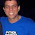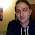## Saturday, September 4, 2010

### The Snail in Golang

It's been some time since I last played with Golang so I decided to spend a bit of time getting to actually know golang by solving the classic snail problem, that is, to write a sequence of numbers in a square matrix of a given size n. Let's say we have a program called `snail`, running it with a number argument (for the square size) should look like this:

```\$ ./snail 5
1  2  3  4  5
16 17 18 19  6
15 24 25 20  7
14 23 22 21  8
13 12 11 10  9
```

So, the solution is straightforward: do alternating row and column sweeps, from the outside going inwards, while incrementing the value to be written into a matrix element.

But first, we have to be able to get the input size of the matrix. For that we'll use the `flag` and `strconv` packages. For the print out, we'll use the `fmt` package. Here's what I came up with.

```package main

import (
"fmt"
"flag"
"strconv"
)

func main() {
// Determine the matrix size, n.
var n int = 1
flag.Parse()
if len(flag.Args()) > 0 {
n, _ = strconv.Atoi(flag.Arg(0))
}
if n < 1 {
n = 1
}

// No need to be complicated for n=1
if n == 1 {
fmt.Println(n)
return
}

// Create the matrix
values := make([][]int, n)
for i := 0; i < n; i++ {
values[i] = make([]int, n)
}

// Fill the matrix with values
avalue := 0
for ifill := 0; ifill < n; ifill++ {
fstart := ifill
fend := n-ifill

// upper x forward sweep
for ixf := fstart; ixf < fend-1; ixf++ {
avalue++
values[fstart][ixf] = avalue
}

// right y forward sweep
for iyf := fstart; iyf < fend-1; iyf++ {
avalue++
values[iyf][fend-1] = avalue
}

// lower x backward sweep
for ixb := fend-1; ixb > fstart; ixb-- {
avalue++
values[fend-1][ixb] = avalue
}

// left y backward sweep
for iyb := fend-1; iyb > fstart; iyb-- {
avalue++
values[iyb][fstart] = avalue
}
}

// Take care of the last value for odd n
if avalue < n*n {
avalue++
values[n/2][n/2] = avalue
}

// Ensure the same spacing for each element
places := int(n*n/10)
entry_width := 1
for places > 0 {
entry_width++
places /= 10
}
format := fmt.Sprintf("%%%dd ", entry_width)

// Print out the snail
for i := 0; i < n; i++ {
for j := 0; j < n ; j++ {
fmt.Printf(format, values[i][j])
}
fmt.Println("")
}

}
```

What about you? Have you tried solving this problem in golang? How would you do it better?

1.can't this be solved as a sequence? i.e 1, 2, 3, 4, 5, 16, 17, 18, 19, 6, etc.

2.hrrmmmm... it's possible but not easy. a good mathematical exercise :-)

3.OOP style(pretty bad one)
http://pastebin.com/raw.php?i=TxQkUbWd

4.A somewhat obscure version using image.Point and image.Rectangle:
http://pastie.org/1503814

5.Here's my version (ran across your page while google surfing)

http://pastebin.com/TePiJYAt

6.I've made this for Project Euler (not exactly the same): https://github.com/franciscosouza/euler/blob/master/problem_028/28.go#L119

7.Here's a version that uses a function to calculate each element based on the size of the grid and its position in it, rather than traversing a multidimensional array in Worm order. It uses two flags, -n and -m to specify the size of the grid.

http://pastie.org/2300048

8.//Thanks for getting me to write my first go program:

package main

import (
"fmt"
"flag"
"strconv"
"math"
)

func main() {
var n int
flag.Parse()
if len(flag.Args()) > 0 {
n,_ = strconv.Atoi(flag.Arg(0))
}

values := make([]int, n*n)

position:=0; s:=0; l:=2*n-1; m:=0
for value := 0; value < n*n; value++ {
values [ position ] = value + 1
m+=2
if m >= l { s++; m=0; l-- }
if s % 2 == 0 {
position += 1 - s % 4
} else {
position += n * ( 2 - s %4 )
}
}

format := fmt.Sprintf("%%%dd ", int ( math.Log ( float64( n * n ) ) / math.Ln10 ) + 1)

for i := 0; i < n*n; i++ {
fmt.Printf(format, values[i])
if ( i + 1 ) % n == 0 {
fmt.Println("")
}
}
}

9.I took a stab at writing something clean using some of my favorite Go idioms. I exploit the pattern in the spiral's side lengths. I think the ideas have already been shown, mostly. And, it's not very concise. But it's nice and readable.

https://github.com/bmatsuo/snail/blob/master/snail.go

Note: I made sure that the output of my program is always flush-left with no excess space on the right. Many of the solutions posted begin to show a gap on the left side of the matrix when the sides are longer than 10.

10.@Gabriel: Your code works great. Being an amateur programmer (using my skills mainly for scientific programming) I am wondering what the m variable is doing since its switching between value 0 and 2 in such a way that the if m >= 1 condition is always true. I tried removing it and obviously the indexing went out of bounds. I am just not figuring out how m is controlling that.